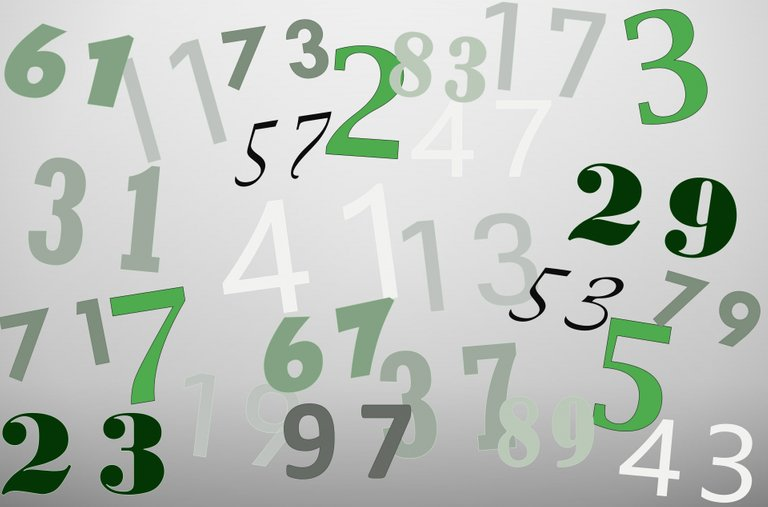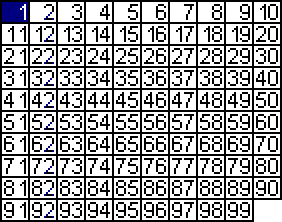# Mathematics - Number Theory - Prime Numbers

in STEMGeeks7 months ago[Image 1]

## Introduction

Hey it's a me again @drifter1!

Today we continue with Mathematics, and more specifically the "Number Theory" series, in order to get into Prime Numbers.

So, without further ado, let's get straight into it!

## Prime Numbers

A prime number is a natural number greater than 1 which is only divisible by 1 and itself. In other words, the only positive integer divisors of a prime number are 1 and itself.

For example, 7 is a prime number because it's only divisible by 1 and 7.

The first 10 prime numbers are:

``````2, 3, 5, 7, 11, 13, 17, 19, 23, 29.
``````

All natural numbers except 1, which is known to be a unique number, can either be prime or not. 1 has exactly 1 factor, any prime has exactly 2 factors and non-primes have more than 2 factors.

Additionally, let's not forget to mention as well, that the only even prime number is 2, as all other even numbers are composite numbers which include 2 in their product representation. So, all prime numbers except 2 are odd numbers.

## Composite Numbers

Any natural number which is not a prime is called a composite number. This basically means that it can be expressed as a product of other numbers, and more specifically by a product of prime numbers (related to the factorization we mentioned last time).

For example, 12 is a composite number as it has the following positive divisors: 1, 2, 3, 4, 6, 12. From these divisors only 2 and 3 are primes. 4 can be expressed as 22 and 6 as 2 × 3. In the end, 12 is basically just 22 × 3, a composition of prime numbers.

## Co-Prime Numbers

Two numbers are called co-primes when they have no common factor apart from 1. When considered in pairs all primes are thus co-primes.

It's easy to check if two composite numbers are co-primes. One simply has to find the Greatest Common Divisor (GCD), also known as Greatest Common Factor (GCF), which has to be 1.

For example, 42 and 55 are co-primes as the GCD = 1.

Explanation:

42 can be expressed by the following product of primes:

``````2 x 3 x 7
``````

whilst 55 by:

``````5 x 11
``````

So, the only common factor is 1.

## Prime Test - Sieve of Eratosthenes

So, how does one test if a number is a prime or not?

If the numbers are small it's easy to do so by applying divisibility rules. But, when the numbers are large, a solution seems to be impossible at first glance.

Fortunately for us, there exists a simple theorem which can be proven easily be contradiction, which states that:

``````If n is a composite number, then it must be divisible by a prime p ≤ √n
``````

In other words, if we want to check the primes up to 100, we only have to check for the primes number in the range up to 10. Thus, we simply have to eliminate the multiples of 2, 3, 5 and 7.

This process is known as the Sieve of Eratosthenes.

Below a GIF, which shows how the process eliminates the non-primes up to 100.[Image 2]

It's easy to see that all even numbers except 2 are eliminated first, followed by all multiples of 3, then all numbers which end on digit 5, and in the end the multiples of 7 as well, leaving us with the first 25 prime numbers, which are:

``````2, 3, 5, 7, 11, 13, 17, 19, 23, 29,
31, 37, 41, 43, 47, 53, 59, 61, 67, 71,
73, 79, 83, 89, 97.
``````

Of course, there are more ways to prime test, but the introduction of additional concepts is needed for that. Thus, theorems such as Fermat's primality test (also known as Fermat's little theorem) will be covered a little later...

## RESOURCES:

### References

Block diagrams and other visualizations were made using draw.io.

## Final words | Next up

And this is actually it for today's post!

Not sure about the next article's topic yet.

See ya!Keep on drifting!

Posted with STEMGeeks

Sort:

Nice explanation drifter.
Keep drifting.

7 months ago

Interesting. I have only ever tried to memorize prime numbers up to 100 instead.

!discovery 37This post was shared and voted inside the discord by the curators team of discovery-it